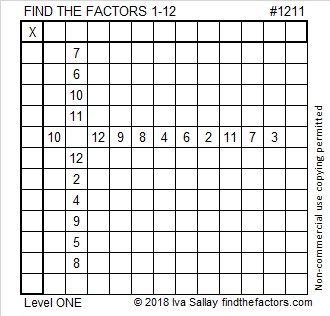# 1211 and Level 1

Today we are reminded that the world can be a very complicated place. Today’s puzzle isn’t the least bit complicated. Just write the numbers from 1 to 12 in the first column and the top row so that the puzzle looks like a multiplication table (but with the factors not in their usual places.) Afterward, you can fill in the rest of the table. You can do this!Print the puzzles or type the solution in this excel file: 12 factors 1211-1220

Now I’d like to share some information about the number 1211:

• 1211 is a composite number.
• Prime factorization: 1211 = 7 × 173
• The exponents in the prime factorization are 1 and 1. Adding one to each and multiplying we get (1 + 1)(1 + 1) = 2 × 2 = 4. Therefore 1211 has exactly 4 factors.
• Factors of 1211: 1, 7, 173, 1211
• Factor pairs: 1211 = 1 × 1211 or 7 × 173
• 1211 has no square factors that allow its square root to be simplified. √1211 ≈ 34.799431211 is the sum of the seventeen prime numbers from 37 to 107.
It is also the sum of seven consecutive primes:
157 + 163 + 167 + 173 + 179 + 181 + 191 = 1211

1211 is the hypotenuse of a Pythagorean triple:
364-1155-1211 which is 7 times (52-165-173)

This site uses Akismet to reduce spam. Learn how your comment data is processed.